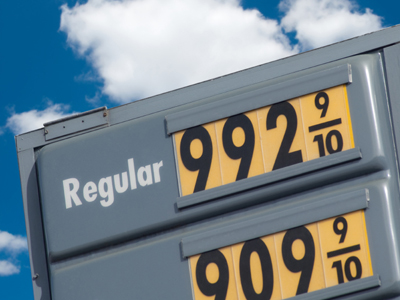992.9 is the same as 992910

# Place Value (Year 6)

By the time children have reached Year Six in KS2, they should be quite comfortable when dealing with place values in Maths. By now they should be well aware of how the values of digits change depending on their position in a number, and know their equivalent values. They should also know the positions after a decimal point - tenths, hundredths and thousandths. They will now be introduced to place holders and should know how the position of digits move when multiplied or divided by 10, 100 or 1,000.

We know the value of a digit changes with its position in a number relative to the decimal point. Before the decimal we have ones, tens and hundreds, and after we have tenths, hundredths and thousandths. We also use place holders. Zeros at the end of a number are place holders. They come after another number, a 1 for example, to show whether it is worth 10, 100 or 1,000.

Take this quiz to discover what you know about place value. This quiz is intended for children aged 10-11.

1.
Which is the number that is equivalent to one hundred and forty two point eight seven?
14287
142.87
1,428.7
142,087
142.87 has 1 hundred
4 tens
2 ones
8 tenths
and 7 hundredths
2.
Which digit is a place holder?
0
1
2
9
0s at the start or end of a number are place holders
3.
When 59,762 is divided by 10, what will we get?
59,7620
5,976.00
5,976.2
5,976,200
To divide by 10 move the numbers one place to the right
4.
Which is the smallest of these numbers?
45 tenths
45 tens
45 hundredths
45 ones
45 hundredths is equal to 0.45
5.
What does the digit 3 in 62.13 represent?
3 hundredths
3 tenths
3 ones
3 tens
Numbers to the right of a decimal point represent 10ths 100ths 1,000ths 10,000ths etc...
6.
When 24,597 is multiplied by 100, what will we get?
245.97
245,970
2,459.7
2,459,700
To multiply by 100 move the numbers two places to the left
7.
What is the value of the digit 9 in 128.96?
9 hundredths
9 tenths
9 ones
9 tens
The first column after the decimal point is tenths so the 9 = 910
8.
When we multiply a digit by ten what should we move?
We should move the digits one place to the left
We should move the digits one place to the right
We should move the digits ten places to the left
We should move the digits ten places to the right
So 300 x 10 = 3,000
9.
When we divide a number by one hundred what should we move?
We should move the digits one place to the left
We should move the digits one place to the right
We should move the digits two places to the left
We should move the digits two places to the right
So 300 divided by 100 = 3
10.
What needs to be added to 45.612 to make 45.682?
7
0.7
0.007
0.07
The 1 and the 8 are both in the hundredths column so 7100 or 0.07 must be added